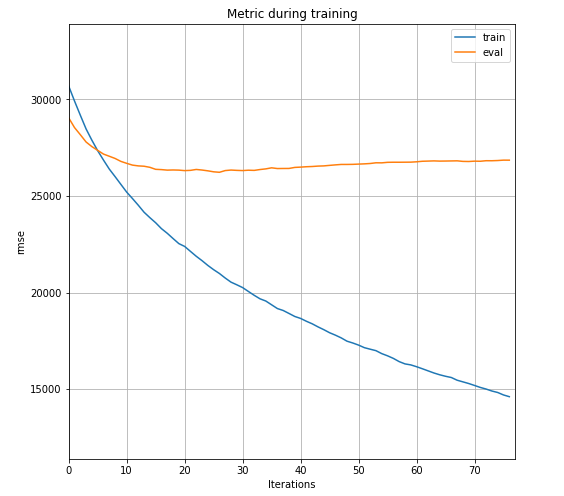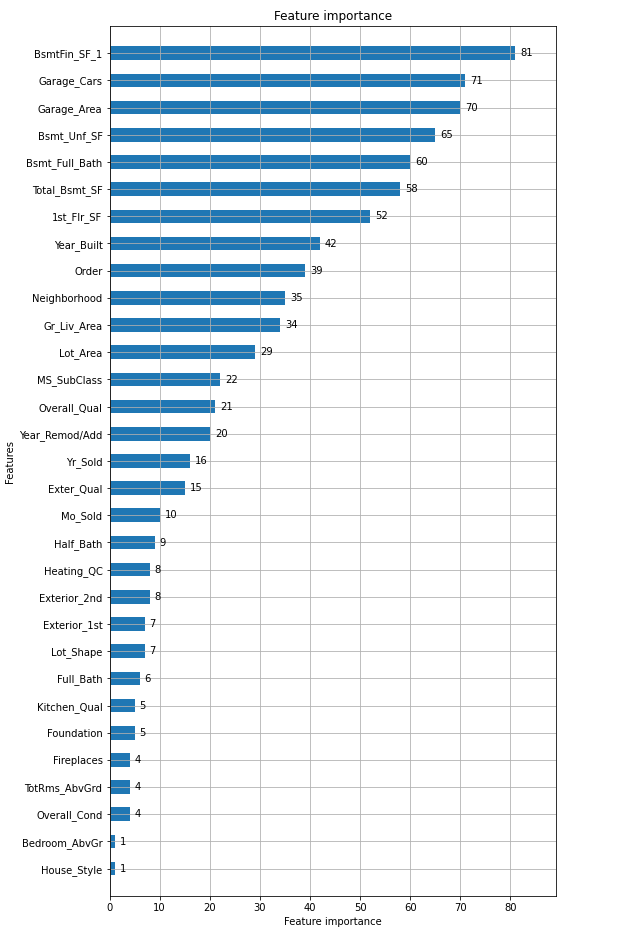# Introduction

Competition for Bigginer held at SIGNATE [5th _Beginner Limited Competition] House Price Forecast in Ames, USA, so I will write a record of participation.

Part 2

Since the result came out, I will add it at the beginning. RMSE: 26106.3566493 Rank: 27/582, so it was the top 4.6%.

--Purpose: Predict the housing price'Sale Price' based on information about the housing and surrounding environment of the city Ames. (Common guy) --Period: 2020/12/01 ~ 2021/01/10
The ranking etc. are listed after that, but it is undecided because the competition is being held. --Only SIGNATE title Beginer can participate (for beginners)

### Competition experience

--Kaggle's Titanic Survivor Prediction only

### policy

--The algorithm uses LightGBM.
It is said that it is often used in competitions, so I'm thinking of trying it once.
I will not do my best until the ensemble with XGBoost. ――Originally, you should understand the data before proceeding, but
First, I created a model with LightGBM in one shot, and based on that, I wrote it in the flow of understanding the data again. ――I think that you can find out as many solutions as you can, so I will do my best without investigating.

# Environment

I installed Ubuntu on WSL2 of Windows10 and tried it mainly with the following libraries.

Library version Remarks
Python 3.8.5 -
lightgbm 3.1.1 -
notebook 6.0.3 jupyter-notebook
scikit-learn 0.24.0 -
matplotlib 3.3.3 -
seaborn 0.11.1 -
optuna 2.3.0 -

# What I did 1

``````train_data = pd.read_csv('./train.csv')
``````

Number of data: 3000 Number of columns: 47 (including objective variable'Sale Price')

#### 1-2. Conversion to categorical data

If you continue learning as it is, an error will occur in the object type (character string), so I converted it to the pandas category type.

``````category_columns = ['MS Zoning', 'Lot Shape',abridgement]  # object->list you want to be in category
for col in category_columns:
train_data[col] = train_data[col].astype('category')

print(train_data.dtypes)
index                int64
Order                int64
MS SubClass          int64
MS Zoning         category
...Abbreviation
``````

The categorical data when I studied before I was a little impressed that I needed One Hot encoding/label encoding, but I could convert it in one shot. It seems that it does not correspond (?) If the version of lightgbm is old, Some pages use label encoders, etc., depending on the URL that is searched.

#### 1-3. Data division/dataset creation

It is divided into 7: 3 by the holdout method and used for learning and verification. Create a dataset for LightGBM with that flow.

``````train, test = train_test_split(train_data, test_size=0.3, shuffle=True, random_state=0)

Y_train = train.loc[:, 'SalePrice']
X_train = train.drop(['index', 'SalePrice'], axis=1)

Y_eval = test.loc[:, 'SalePrice']
X_eval = test.drop(['index', 'SalePrice'], axis=1)

lgb_train = lgb.Dataset(X_train, Y_train)
lgb_eval = lgb.Dataset(X_eval, Y_eval, reference=lgb_train)
``````

I deleted the index somehow, but it's LightGBM and maybe it wasn't necessary.

#### 1-4. Learning

Since it is a trial, only the minimum settings are required.

``````lgbm_params = {
'boosting_type': 'gbdt',
'objective': 'regression',
'metric': 'rmse',
'max_depth' : 10
}

evals_results = {}
model = lgb.train(lgbm_params, lgb_train,
num_boost_round=1000,
valid_sets=[lgb_eval, lgb_train],
valid_names=['eval', 'train'],
early_stopping_rounds=50,
evals_result=evals_results,
verbose_eval=50)
``````

# Result 1

### Learning curve

Show learning curve with plot_metric () of lightgbm https://lightgbm.readthedocs.io/en/latest/pythonapi/lightgbm.plot_metric.html

``````lgb.plot_metric(evals_results, metric='rmse', figsize=(8, 8))
``````It peaked after 27 learnings, after which the performance slightly decreased due to overfitting. It seems that overfitting can be suppressed a little more by parameter tuning.

Note: The first argument of plot_metric () is

Dictionary returned from lightgbm.train()

I put the return value model of train () because it was in the official Document, but it was useless ... When Dict is set in the evals_result parameter of train () Since it outputs the learning result, it seems that it is necessary to set it.

importance Display the importance of features with plot_importance () of lightgbm https://lightgbm.readthedocs.io/en/latest/pythonapi/lightgbm.plot_importance.html

``````lgb.plot_importance(model, height=0.5, figsize=(8, 16))
``````BsmtFin SF 1 (underground area) and Garage xxx (car storage) are important. I felt like I understood the essence of the data.

### Current ranking

RMSE: 26818.0705594 Ranking: 132/542, so the top 25%

# What I did 2

I replaced it with cross-validation because I wanted to make it a little more versatile. LightGBM has a cv () function, so it was possible by almost replacing the train () function. Depending on the URL that you search for, there is a way to implement it yourself with StratifiedKFold (), which was confusing, but He explained it nicely on the following page. https://blog.amedama.jp/entry/lightgbm-cv-implementation

``````lgb_train = lgb.Dataset(X_train, Y_train)
# lgb_eval = lgb.Dataset(X_eval, Y_eval, reference=lgb_train)

lgbm_params = {
'boosting_type': 'gbdt',
'objective': 'regression',
'metric': 'rmse',
'max_depth' : 10
}

model = lgb.cv(lgbm_params, lgb_train,
num_boost_round=1000,
early_stopping_rounds=50,
verbose_eval=50,
nfold=7,
shuffle=True,
stratified=False,
seed=42,
return_cvbooster=True,
)
``````

Note: Setting the return_cvbooster parameter to True makes model ['cvbooster'] a list of training models. You can use it to predict (). It seems that return_cvbooster does not support old LightGBM, and it may not be used if it is a URL that is searched.

# Result 2

### Current ranking by cross-validation

RMSE: 26552.7208586 Ranking: 86/543, so the top 16%

RMSE improved by about 250. It seems that the division of train_test_split () was not good. Ranking improved by about 10%.

# Summary 1

It is a competition only for beginners, but it seems that it will be in the top 16% just by using LightGBM. From here, try data understanding and parameter adjustment. Part 2